### 13.2.4.1 Better unicycle models

Improvements to the models in Section 13.1 can be made by placing integrators in front of action variables. For example, consider the unicycle model (13.18). Instead of directly setting the speed using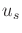, suppose that the speed is obtained by integration of an action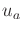that represents acceleration. The equation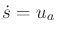is used instead of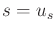, which means that the action sets the change in speed. Ifis chosen from some bounded interval, then the speed is a continuous function of time.

How should the transition equation be represented in this case? The set of possible values forimposes a second-order constraint onand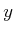because double integration is needed to determine their values. By applying the phase space idea,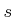can be considered as a phase variable. This results in a four-dimensional phase space, in which each state is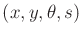. The state (or phase) transition equation is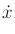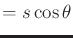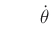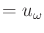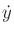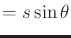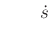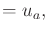(13.45)

which should be compared to (13.18). The actionwas replaced bybecause now speed is a phase variable, and an extra equation was added to reflect the connection between speed and acceleration.

The integrator idea can be applied again to make the unicycle orientations a continuous function of time. Let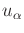denote an angular acceleration action. Let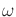denote the angular velocity, which is introduced as a new state variable. This results in a five-dimensional phase space and a model called the second-order unicycle: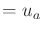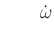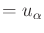(13.46)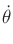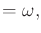in which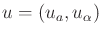is a two-dimensional action vector. In some contexts,may be fixed at a constant value, which implies thatis fixed to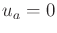.

Steven M LaValle 2020-08-14